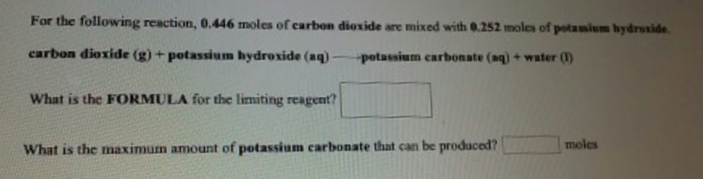# Problem: For the following reaction, 0.446 moles of carbon dioxide are mixed with 0.252 moles of potassium hydroxide.carbon dioxide (g) + potassium hydroxide (aq) → potassium carbonate (aq) + water (l) What is the FORMULA for the limiting reagent? What is the maximum amount of potassium carbonate that can be produced?

###### FREE Expert Solution
79% (293 ratings)###### Problem Details

For the following reaction, 0.446 moles of carbon dioxide are mixed with 0.252 moles of potassium hydroxide.

carbon dioxide (g) + potassium hydroxide (aq) → potassium carbonate (aq) + water (l)

What is the FORMULA for the limiting reagent?

What is the maximum amount of potassium carbonate that can be produced?